Rigid Pavement

# Rigid Pavement - Notes | Study Transportation Engineering - Civil Engineering (CE)

 Table of contentsIntroduction1 Crore+ students have signed up on EduRev. Have you?

Introduction

Rigid pavements are those which posses note worthy flexural strength or flexural rigidity. The stresses are not transferred from grain to grain to the lower layers as in the ease of flexible pavement layers. The rigid pavements are made of Portland cement concrete-either plain, reinforced or prestressed concrete. The plain cement concrete slabs are expected to take-up about 40 kg/cm2 flexural stress.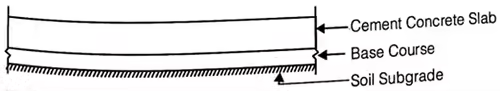(i) Modulus of subgrade reaction (k),
k = P/Δ
where, k = Modulus of subgrade reaction (kg/cm2/cm)
p = Pressure required for ‘Δ’ deflection (kg/cm2)
Δ = Deflection(cm)
For 75 cm dia plate, Δ = 1.25 mm

(ii) Radius of Relative Stiffness (l)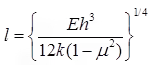where, l = Radius of relative stiffness, cm
E = Modulus of elasticity of cement concrete (kg/cm2)
μ = Poisson’s ratio for concrete = 0.15
h = Slap thickness (cm)

(iii) Equivalent Radius of Resisting Section (b)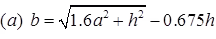when a < 1.724 h
(b) b = a when a > 1.724h
where, a = Radius of contact area (cm)
h = Slab thickness (cm)

(iv) Glodbeck’s Formula for Stress due to Corner Load
SC = 3P/h2
where, SC = Stress due to corner load (kg/cm2)
h = Thickness of slab (cm).

(v) Westergards Stress Equation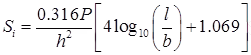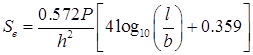where, h = Slab thickness (cm)
a = Radius of contact area (cm)
l = Radius of relative stiffness (cm)
b = Radius at resisting section (cm).

(vi) Warping Stresses
(a) Stress at Interior Region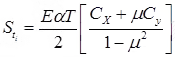where,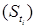Warping stress at interior region (kg/cm2)
E = Modulus of elasticity of concrete, (kg/cm2)
α = Coefficient of thermal expansion (/°c)
CX = Coefficient based on (Lx/l) in desired direction.
Cy = Coefficient based on (Ly/l) in right angle to the above direction.
μ = Poissons’s ratio ∼ 0.15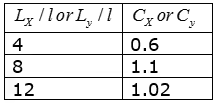LX & Ly are the dimensions of the slab considering along X & y directions along the length & width of slab.
(b) Stress at Edge Region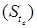(c) Stress Corner Region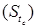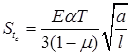where, a = Radius of contact area
l = Radius of relative stiffness

(vii) Frictional Stress (Sf)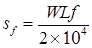where, SF = Frictional stress (kg/cm2)
W = Unit weight of concrete, (kg/cm3)
f = Friction constant or coefficient of subgrade restraint
L = Slab length (m)
B = Slab width (m)

(viii) Combination of Stresses

1. Critical Combination During Summer
(a) Stress for edge/interior regions at Bottom = (+ load stress) + (warping stress of day time) – Frictional stress
(b) Stress for corner region at top = (+ load stress + warping stress at night)
2. Critical Combination During Winter
(a) Stress for edge/interior at bottom = (+ load stress + warping stress at day time + Frictional stress)
(b) Stress for corner at top = (load stress + warping stress at night)

Design of Joints in Cement Concrete Pavements
(i) The spacing of expansion joints, (Le)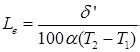Where, δ’ = Maximum expansion in slab (cm)
Le = Spacing of expansion joint (m)
α = Coefficient of thermal expansion of concrete (/°c)

(ii) The spacing of the contraction joint, (Lc)
(a) When reinforcement is not provided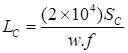where, Lc = Spacing of contraction joint (m)
SC = Allowable stress in tension in cement concrete.
f = Coefficient friction ∼ 1.5
w = Unit weight of cement concrete (kg/m3).
(b) When reinforcement is provided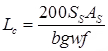where, SS = Allowable tensile stress in steel (kg/cm2)
1400kg/cm2
AS = Total area of steel in cm2.

(iii) Longitudinal Joints

(a)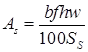where, AS = Area of steel required per meter length of joint (cm2)
b = Distance between the joint & nearest free edge (m)
h = Thickness of the pavement (cm)
f = Coeff. of friction ∼ 1.5
w = Unit wt. of concrete (kg/cm3)
Ss = Allowable working stress in tension for steel (kg/cm2)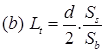where, Lt = Length of tie bar
SS = Allowable stress in tension (kg/cm2) ∼ 1400
Sb = Allowable bond stress in concrete (kg/cm2)
= 24.6 kg/cm2 for deformed bars
= 17.5 kg/cm2 for plain tie bars
d = diameter of tie bar (cm).

IRC Recommendations for Design of Cement Concrete Pavements

Ad = P’[1 + r](n + 20)
where, Ad = Number of commercial vehicles per day (laden weight > 3 tonnes)
P’ = Number of commercial vehicles per day at last count.
r = Annual rate of increase in traffic intensity.
n = Number of years between the last traffic count & the commissioning of new cement concrete pavement.

The document Rigid Pavement - Notes | Study Transportation Engineering - Civil Engineering (CE) is a part of the Civil Engineering (CE) Course Transportation Engineering.
All you need of Civil Engineering (CE) at this link: Civil Engineering (CE)

## Transportation Engineering

22 videos|68 docs|39 tests
 Use Code STAYHOME200 and get INR 200 additional OFF

## Transportation Engineering

22 videos|68 docs|39 tests

Track your progress, build streaks, highlight & save important lessons and more!

,

,

,

,

,

,

,

,

,

,

,

,

,

,

,

,

,

,

,

,

,

;